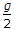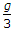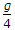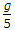# Civil Engineering - Applied Mechanics - Discussion

### Discussion :: Applied Mechanics - Section 1 (Q.No.21)

21.

If the tension in a cable supporting a lift moving upwards is twice the tension when the lift is movng downwards, the acceleration of the lift, is

 [A].[B].[C].[D].Explanation:

No answer description available for this question.

 M Zafar said: (Apr 5, 2015) Any one explain how?

 Jay Bermudo said: (May 12, 2015) If you look closely, there are three cables in the problem. It says the word "lift" three times so the cable is three. So gravity divided by three is the answer.

 Amr said: (Jul 19, 2015) According to motion equation sum of forces = mass*acceleration (a). In case going down force up is tension t and down is weight mass*g. So m*g-t = m*a. So t = m*g-m*a....(1). In case of going up force up is 2*t and force down is m*g. So 2*t-m*g = m*a. So from 1 t = m*g-m*a then 2*m*g-2*m*a-m*g = m*a. Then m*g-2*m*a = m*a. So m*g = 3*m*a. So g = 3 a. So a = g/3.

 Kiran said: (May 21, 2017) Yes, your explanation is correct @Amr.

 Suneel Kurmi said: (May 30, 2017) Tension in the cable. Moving downward T= m(g-a). Upward T=m(g+a). B option is correct.